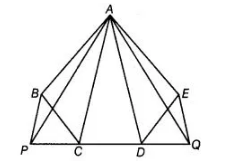# n figure, ABCDE is any pentagon.`
Question:

n figure, ABCDE is any pentagon. BP drawn parallel to AC meets DC produced at P and EQ drawn parallel to AD meets CD produced at Q. Prove that ar (ABCDE) = ar (ΔAPQ).Solution:

Given ABCDE is a pentagon.

BP || AC and EQ|| AD.

To prove ar (ABCDE) = ar (APQ)

Proof We know that, triangles on the same base and between the same parallels are equal in area.

Similarly, ΔACP and ΔACB lie on the same base AC and between the same parallels AC and BP.

So, ar (ΔACP) = ar (ΔACB) …(ii)

On adding Eqs. (i) and (ii), we get